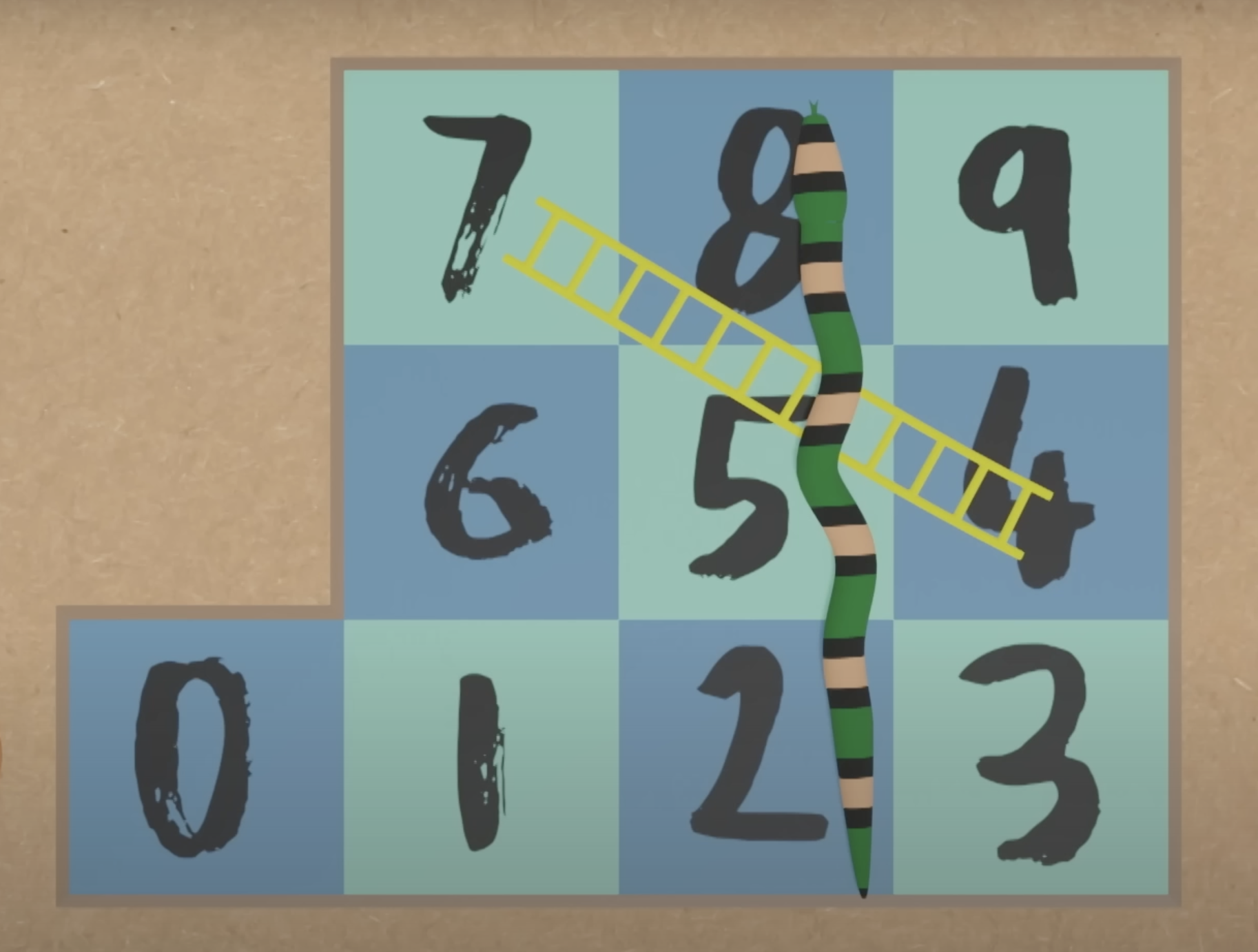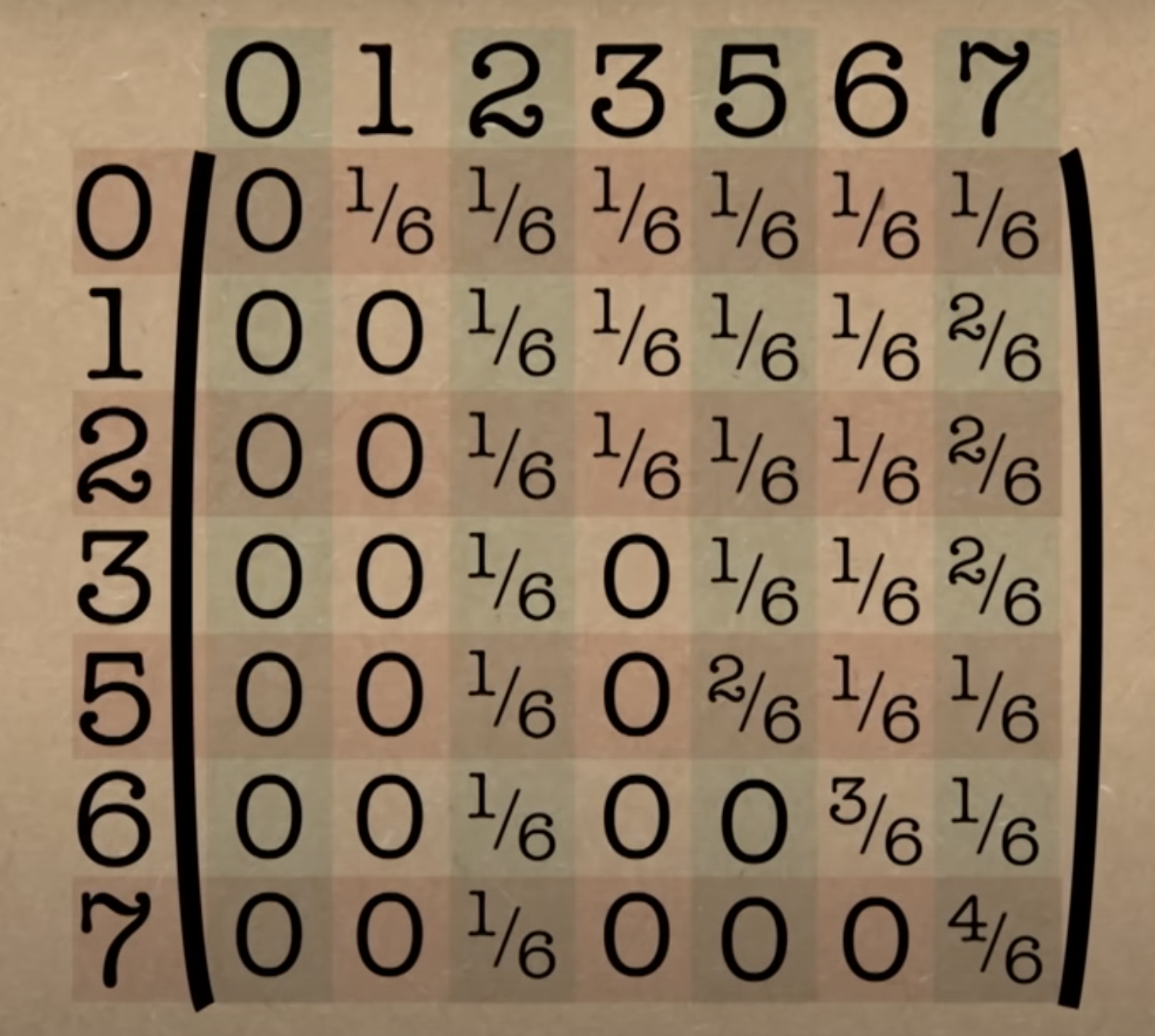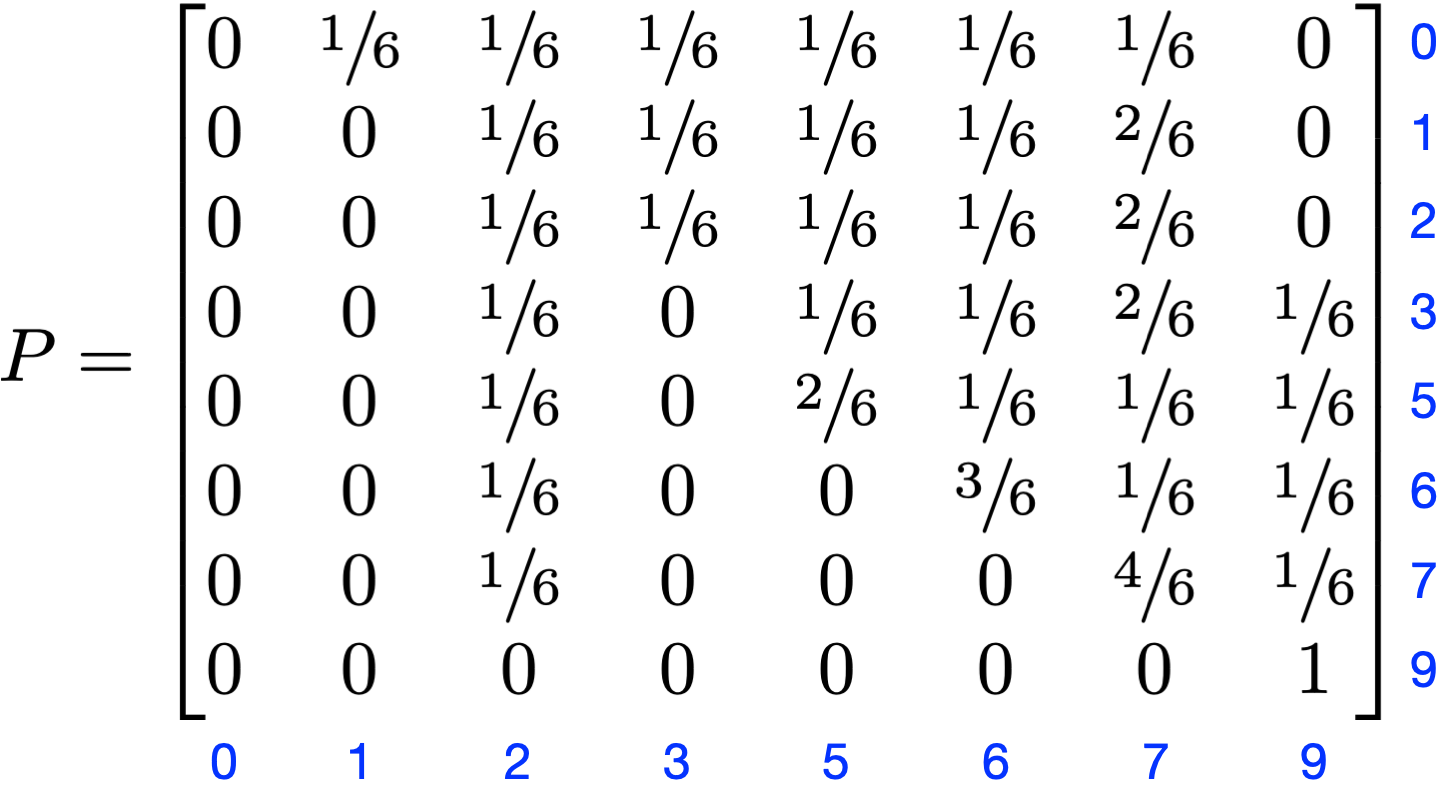This week’s Numberphile video features the BBC’s favorite mathematician, Marcus du Sautoy, who explains how the game Snakes and Ladders1 is governed by the mathematics of Markov chains. Despite some experience analyzing Markov chains in grad school, I had trouble understanding one part of the video, so I pulled out my old textbook to clear things up.

But before we get into the math, a short digression. One of my favorite episodes of Melvin Bragg’s In Our Time radio show was the “Zeno’s Paradoxes” show from back in 2016. I distinctly remember being infuriated by the show because du Sautoy’s fellow guests, a philosopher and a classicist, clearly thought that Zeno’s reasoning on paradoxes like Achilles and the tortoise and the flying arrow was still valuable. Not because it gives us insight into the historical development of thinking on infinite series or state space representation—which it absolutely does—but because it really is puzzling that Achilles passes the tortoise. What made the show so entertaining was listening to du Sautoy’s obvious frustration with what he considered their obtuseness with regard to solved problems and his need to suppress that frustration for the sake of politeness.

OK, onto the math. Du Sautoy starts by setting up a small Snakes and Ladders game with just ten squares—labeled 0 through 9—one ladder, and one snake.You move across the board by rolling a single die and advancing your marker accordingly. The goal is to reach Square 9 with an exact roll. If you roll a number that is more than you need to reach Square 9, you stay where you are until the next roll.

He then goes through the construction of a transition matrix, in which each element is the probability of moving from the square represented by the row to the square represented by the column. It looks like this when he’s done:The row and column of numbers outside the matrix are the squares on the game board. You’ll note that three of the positions are missing:

• Square 4 is missing because you never stay on it. If you land there, you immediately climb up to Square 7 via the ladder.
• Similarly, Square 8 is missing because if you land on it, you immediately slide down to Square 2 via the snake.
• Square 9 is missing because, du Sautoy says, the game is over when you reach that square. While that’s certainly true—and we’ll see later that entries for row and column 9 aren’t used in the calculations—I still think it’s helpful to include Square 9 in the transition matrix. Like this:I’m calling this matrix $P$ to avoid confusing it with du Sautoy’s transition matrix, which he calls $Q$. We’ll return to this matrix soon.

It’s after construction his transition matrix that du Sautoy does what I don’t understand. He wants to calculate the expected number of turns it will take to reach Square 9 from Square 0. The equation he comes up with is this infinite sum,

$I+Q+Q2+Q3+Q4+…I + Q + Q^2 + Q^3 + Q^4 + \ldots$

where $I$ is the identity matrix and the powers represent matrix multiplication. The sum of the top row of the resulting matrix is the expected number of rolls to get to Square 9. I understand everything du Sautoy says as he explains what each of the terms in this series is, but I don’t get why it leads to the expected number of rolls.

I even understand the next part of the video, where some clever algebra shows that this infinite series converges to the non-infinite matrix2

$(I−Q)−1\left( I - Q \right)^{-1}$

So the sum of the elements of the top row of this inverted matrix is the expected number of rolls to get to Square 9.

As it happens, you can get to this final answer by a method I understand. We’ll start by returning to the $P$ matrix I defined earlier,As you can see, the first seven rows and columns are the same as $Q$. The first seven elements of the last column consists of the probabilities of getting to Square 9 from each of the earlier squares. These are the probabilities that were skipped over in the video, and they’re all either zero or $1/6$ because the roll has to be exactly the number of squares away from 9 you are. Note also that the sum of all the terms in each row must be 1 because you have to land somewhere after each row.

The last row is special. It says that once you’re on Square 9, you can’t go anywhere else. In the study of Markov chains, this is known as an absorbing state, and the properties of absorbing states are pretty well known. In particular, there’s an established formula for the expected number of rolls to get from Square $i$ to Square 9, the absorbing state.3

We’ll call the expected number of rolls to get from Square $i$ to Square 9 ${m}_{i}$. One thing we can say right away is that

$m9=0m_9 = 0$

because you don’t need to roll to get to the end when you’re already there. For all the other values of $i$, we use some lateral thinking.

First, we know that if we’re on Square 1, it takes ${m}_{1}$ rolls to get to the end—that’s by definition. So if we start on Square 0 and go to Square 1 on our first roll, it will take, on average, $1+{m}_{1}$ rolls to get to the end. But of course we may not go from Square 0 to Square 1; we might go to Square 2 or 3 or whatever. Each of these possibilities for the first roll has a probability assigned to it in the transition matrix, $P$. So the expected value of the number of rolls to get from Square 0 to Square 9 is

$m0=(1+m1)p01+(1+m2)p02+(1+m3)p03m_0 = (1 + m_1)\,p_{01} + (1 + m_2)\,p_{02} + (1 + m_3)\,p_{03}$ $+(1+m5)p05+(1+m6)p06+(1+m7)p07\quad + \:(1 + m_5)\,p_{05} + (1 + m_6)\,p_{06} + (1 + m_7)\,p_{07}$

where the $p$ values are taken from the top row of the $P$ matrix. It may look like we’ve gone backwards here, introducing all the other ${m}_{i}$ into an equation for ${m}_{0}$. But let’s see what happens when we generalize this to any starting square and make the equation more compact with the summation symbol.

$mi=∑allj(1+mj)pijm_i = \sum\limits_{\mathrm{all}\: j} (1 + m_j) \; p_{ij}$

Doing some algebra, we get

$mi=∑alljpij+∑alljmjpijm_i = \sum\limits_{\mathrm{all}\: j} p_{ij} + \sum\limits_{\mathrm{all}\: j} m_j \; p_{ij}$

Note that the first sum in this equation is the sum of all the elements of $P$ in row $i$, and that is 1 for each row. Combining that with ${m}_{9}=0$, we can simplify this equation to

$mi=1+∑allj≠9mjpijm_i = 1 + \sum\limits_{\mathrm{all}\: j \neq 9} m_j \; p_{ij}$

This works for all values of $i\ne 9$. So what we have here are seven linear equations in seven unknowns, which is hard to solve by hand, but easy to solve with a computer. If we put it in matrix form, it becomes interesting:

$m=1+Qmm = 1 + Q\, m$

Here, the $m$ is a column vector of all the ${m}_{i}$ terms, the $1$ is a column vector of ones, and $Q$ is the matrix $P$ without its last row and column—yes, it’s du Sautoy’s transition matrix that ignored Square 9. Since $m=I\phantom{\rule{0.167em}{0ex}}m$, we can do the following manipulation:

$m=Im=1+Qmm = I\, m = 1 + Q \, m$ $(I−Q)m=1(I - Q)\, m = 1$ $m=(I−Q)−11m = \left( I - Q \right)^{-1}\, 1$

Well, this should look familiar. Because we’re multiplying by a column vector of ones, each element of $m$ is equal to the sum of the corresponding row of the inverted matrix. And therefore the expected number of rolls to get from Square 0 to Square 9 is the sum of the top row of

$(I−Q)−1\left( I - Q \right)^{-1}$

In solving this problem, you wouldn’t explicitly invert the matrix, because that’s more computationally intensive than solving a set of linear equations. But I wrote it out this way to show that it’s the same result as du Sautoy’s.

One thing that isn’t the same as du Sautoy’s is the numerical answer. I got the following:

$m=[8.68.48.47.27.27.27.2]Tm = [ 8.6 \quad 8.4 \quad 8.4 \quad 7.2 \quad 7.2 \quad 7.2 \quad 7.2 ]^T$

As you can see, my answer for ${m}_{0}$ is 8.6, not 10 as du Sautoy says in the video. I checked my expression for $Q$ and it matched his. I went through all the transition probabilities again and agreed with his. Then I looked in the comments and found that several Numberphile followers also got 8.6, which made me feel better about my answer. While it’s possible we all made the same mistake, given that this is a very simple calculation, I think it’s more likely du Sautoy had a typo somewhere in his work. If you see an error here, though, I’d like to know about it.

1. Probably known to most Americans as Chutes and Ladders, thanks to Hasbro